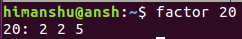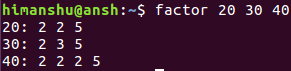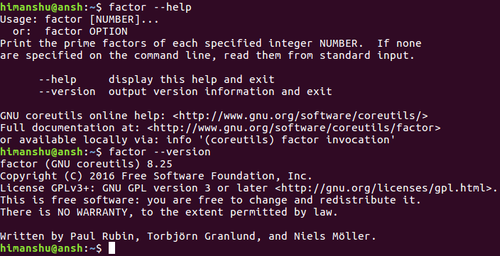# Linux factor command tutorial for beginners (with examples)

### On this page

If you are a Linux command line user, and your work revolves around prime numbers, there's a command line utility that might be of your help. The tool in question is factor, and in this tutorial, we will quickly discuss things like why it exists, how it works, and how you can use it. Please note that all examples and instructions mentioned here have been tested on Ubuntu 16.04LTS.

## Linux factor command

The factor command in Linux gives you prime factors of a number. Here's the syntax of the tool:

``factor [NUMBER]...``

And here's how the utility's man page describes it:

``Print the prime factors of each specified integer NUMBER.  If none are specified on the command line, read them from standard input.``

## Q1. How to use factor command?

Usage is pretty easy (as you'd have guessed through its syntax described above). Just pass the number which you want to factor as an argument to the tool. For example, to find the prime factors of, say, 20, use factor in the following way:

``factor 20``

Following is the output that was produced on my system:So that's how you can use the factor command. Of course, you can pass multiple numbers as argument to the command in one go.The tool doesn't offer any other major command line options, except the regular --help and --version that are there for almost every command line tool in Linux.## Q2. What algorithm factor uses?

As per the GNU documentation, the factor command uses Pollard-Brent rho algorithm. The documentation (see here), also suggests the algorithm is only effective for numbers with relatively small factors. Here's an excerpt from the documentation:

``Factoring large numbers is, in general, hard. The Pollard-Brent rho algorithm used by factor is particularly effective for numbers with relatively small factors. If you wish to factor large numbers which do not have small factors (for example, numbers which are the product of two large primes), other methods are far better. If factor is built without using GNU MP, only single-precision arithmetic is available, and so large numbers (typically 2^{128} and above) will not be supported. The single-precision code uses an algorithm which is designed for factoring smaller numbers.``

## Q3. Why factor exists?

Well, there's no official word on this, but experts speculate someone (or a group) dealing with prime numbers developed the tool over half a century back. And the fact that it has since been included in Unix and Linux distributions can be attributed to lack of any reason to not to do so.

A discussion on this topic can be accessed here.

## Conclusion

Clearly, the factor command is a niche tool. Only those dealing with prime numbers/factors would find it useful. But then again, there's no harm learning about the utility (especially when there's practically no learning curve associated with it) and remembering it, as you never know, when it might save your day.

You can access factor's man page by heading here.view as pdf |print

Add comment